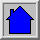## Simple Chaotic Flow GIF Animations

The image above is the solution of one of the algebraically simplest examples of a chaotic flow. It and the 18 other cases listed below turned up in a search of 3-dimensional systems of ordinary differential equations with either 5 terms and 2 quadratic nonlinearities or 6 terms and 1 quadratic nonlinearity. All of these cases are algebraically simpler than the classic Lorenz and Rossler examples, each of which has 7 terms. In each case, the y-axis is upward and the object rotates in the x-z plane. To view these animations, you need a browser that supports animated GIF files. The software that was used to generate the images is available. Case A (shown above) is a conservative system; all others are dissipative.  Case D has the unusual combination of time-reversal invariance and dissipation arising from a symmetric attractor/repellor pair.
• Case A (157,095 bytes) : dx/dt = y, dy/dt = -x + yz, dz/dt = 1 - y2
• Case B (199,857 bytes) : dx/dt = yz, dy/dt = x - y, dz/dt = 1 - xy
• Case C (78,515 bytes) : dx/dt = yz, dy/dt = x - y, dz/dt = 1 - x2
• Case D (77,876 bytes) : dx/dt = -y, dy/dt = x + z, dz/dt = xz + 3y2
• Case E (24,240 bytes) : dx/dt = yz, dy/dt = x2 - y, dz/dt = 1 - 4x
• Case F (80,564 bytes) : dx/dt = y + z, dy/dt = -x + 0.5y, dz/dt = x2 - z
• Case G (57,560 bytes) : dx/dt = 0.4x + z, dy/dt = xz - y, dz/dt = -x + y
• Case H (82,055 bytes) : dx/dt = -y + z2, dy/dt = x + 0.5y, dz/dt = x - z
• Case I (45,318 bytes) : dx/dt = -0.2y, dy/dt = x + z, dz/dt = x + y2 - z
• Case J (37,512 bytes) : dx/dt = 2z, dy/dt = -2y + z, dz/dt = -x + y + y2
• Case K (80,325 bytes) : dx/dt = xy - z, dy/dt = x - y, dz/dt = x + 0.3z
• Case L (47,827 bytes) : dx/dt = y + 3.9z, dy/dt = 0.9x2 - y, dz/dt = 1 - x
• Case M (53,162 bytes) : dx/dt = -z, dy/dt = -x2 - y, dz/dt = 1.7 + 1.7x + y
• Case N (65,440 bytes) : dx/dt = -2y, dy/dt = x + z2, dz/dt = 1 + y - 2z
• Case O (62,420 bytes) : dx/dt = y, dy/dt = x - z, dz/dt = x + xz + 2.7y
• Case P (89,513 bytes) : dx/dt = 2.7y + z, dy/dt = -x + y2, dz/dt = x + y
• Case Q (60,647 bytes) : dx/dt = -z, dy/dt = x - y, dz/dt = 3.1x + y2 + 0.5z
• Case R (102,254 bytes) : dx/dt = 0.9 - y, dy/dt = 0.4 + z, dz/dt = xy - z
• Case S (57,949 bytes) : dx/dt = -x - 4y, dy/dt = x + z2, dz/dt = 1 + x
Ref: J. C. Sprott, Phys. Rev. E 50, R647-R650 (1994)

Since these cases were discovered, an even simpler example of a chaotic flow was found, and it has been rigorously proved that there can be no simpler example of an ordinary differential equation with a quadratic nonlinearity and chaotic solutions.

Most of the above cases have small basins of attraction and are chaotic only over a small region of their parameter space. The different dynamic regions for the cases with six terms and hence two parameters (cases F through S) are shown here.

Electronic circuits corresponding to the above systems have been constructed and animated by Glen K. from Australia as described on his website.Back to Sprott's Fractal Gallery